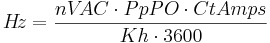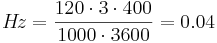## Overview

For some Pulse WattNode® meter applications, you need to specify the pulses per watt-hour or pulses per kWh (kilowatt-hour). The easiest approach is to use Option Kh, but you can use Option Hz=xxx, where xxx is the full-scale pulse frequency for the WattNode meter. This article discusses how to manually compute the correct Option Hz value to get a desired pulses per watt-hour or pulses per kWh (kilowatt-hour) value.

## Computation

• nVAC – This is the nominal line voltage (phase to neutral) of the WattNode model. For delta models, this is a virtual voltage, since there may not be a neutral connection. Note: this is not the actual measured voltage.
WattNode Model nVAC
WNB-3Y-208-P 120
WNB-3Y-400-P 230
WNB-3Y-480-P 277
WNB-3Y-600-P 347
WNB-3D-240-P 120*
WNB-3D-400-P 230*
WNB-3D-480-P 277*

*Note: these are “virtual” phase-to-neutral voltages used for delta model power and energy computations.

• PpPO – “Phases per Pulse Output”. This is the number of meter voltage phases associated with a pulse output channel.
• Standard Bidirectional: PpPO = 3
• Option DPO (Dual Positive Outputs): PpPO = 3
• Option P3 (Per-Phase Outputs): PpPO = 1
• Option PV (Photovoltaic): PpPO = 2 for outputs P1 and P2, PpPO = 1 for output P3
• CtAmps – This is the current transformer (CT) rated amps. Note: If the wires being measured are passed through the CTs more than once, then CtAmps is the rated CT current divided by the number of times that the wire passes through the CT.
• Hz – This is the full-scale pulse frequency of the WattNode Pulse meter.
• Kh – Watt-hours per pulse. Kh / 1000 = kilowatt-hours per pulse.$H!z=frac{nV!AC cdot PpPO cdot CtAmps}{Kh cdot 3600}$

Once you’ve computed the desired full-scale pulse frequency, you can order a WattNode meter with this frequency using Opt Hz=xxx where xxx is the computed value. For example, if you want watt-hours per pulse to be 1000 (1.0 kWh per pulse) for a WNB-3Y-208-P with 400A CTs then:$H!z=frac{120 cdot 3 cdot 400}{1000 cdot 3600}=0.04$

So you should order WNB-3Y-208-P Opt Hz=0.04.